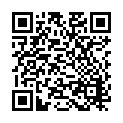Lavoisier S.A.S.
14 rue de Provigny
94236 Cachan cedex
FRANCE

Heures d'ouverture 08h30-12h30/13h30-17h30
Tél.: +33 (0)1 47 40 67 00
Fax: +33 (0)1 47 40 67 02Url canonique : www.lavoisier.fr/livre/mathematiques/applied-regression-analysis-a-research-tool-2nd-ed-1998/descriptif_1277812
Url courte ou permalien : www.lavoisier.fr/livre/notice.asp?ouvrage=1277812

# Applied regression analysis, a research tool, 2nd ed 1998 (2nd Ed., 2nd ed. 1998. Softcover reprint of the original 2nd ed. 1998) A Research ToolSpringer Texts in Statistics Series

Langue : Anglais

## Auteurs : Rawlings John O., Pantula Sastry G., Dickey David A.• ## Commentaire

Least squares estimation, when used appropriately, is a powerful research tool. A deeper understanding of the regression concepts is essential for achieving optimal benefits from a least squares analysis. This book builds on the fundamentals of statistical methods and provides appropriate concepts that will allow a scientist to use least squares as an effective research tool. Applied Regression Analysis serves as an excellent text for a service course on regression for non-statisticians and as a reference for researchers. It also provides a bridge between a two-semester introduction to statistical methods and a thoeretical linear models course. Applied Regression Analysis is aimed at the scientist who wishes to gain a working knowledge of regression analysis. The basic purpose of this book is to develop an understanding of least squares and related statistical methods without becoming excessively mathematical. It is the outgrowth of more than 30 years of consulting experience with scientists and many years of teaching an applied regression course to graduate students. Applied Regression Analysis serves as an excellent text for a service course on regression for non-statisticians and as a reference for researchers. It also provides a bridge between a two-semester introduction to statistical methods and a thoeretical linear models course. Applied Regression Analysis emphasizes the concepts and the analysis of data sets. It provides a review of the key concepts in simple linear regression, matrix operations, and multiple regression. Methods and criteria for selecting regression variables and geometric interpretations are discussed. Polynomial, trigonometric, analysis of variance, nonlinear, time series, logistic, random effects, and mixed effects models are also discussed. Detailed case studies and exercises based on real data sets are used to reinforce the concepts. The data sets used in the book are available on the Internet.
1. Review of Simple Regression, 2. Introduction to Matrices, 3. Multiple Regression in Matrix Notation, 4. Analysis of Variance and Quadratic Forms, 5. Case Study: Five Independent Variables, 6. Geometric Interpretation of Least Squares, 7. Model Development: Variable Selection, 8. Polynomial Regression, 9. Class Variables in Regression, 10. Problem Areas in Least Squares, 11. Regression Diagnostics, 12. Transformation of Variables, 13. Collinearity, 14. Case Study: Collinearity Problems, 15. Models Nonlinear in the Parameters, 16. Case Study: Response Curve Modeling, 17. Analysis of Unbalanced Data, 18. Mixed Effects Models, 19: Case Study: Analysis of Unbalanced Data.
Least squares methods is an essential statistical technique in many areas including the life sciences, environmental sciences, and agriculture. This book provides the background necssary to understand the important concepts of regression analysis without becoming excessively mathematical.

Date de parution :

Ouvrage de 660 p.

17.8x25.4 cm

Disponible chez l'éditeur (délai d'approvisionnement : 15 jours).

94,94 €

### Mots-clés :

En continuant à naviguer, vous autorisez Lavoisier à déposer des cookies à des fins de mesure d'audience. Pour en savoir plus et paramétrer les cookies, rendez-vous sur la page Confidentialité & Sécurité.
FERMER